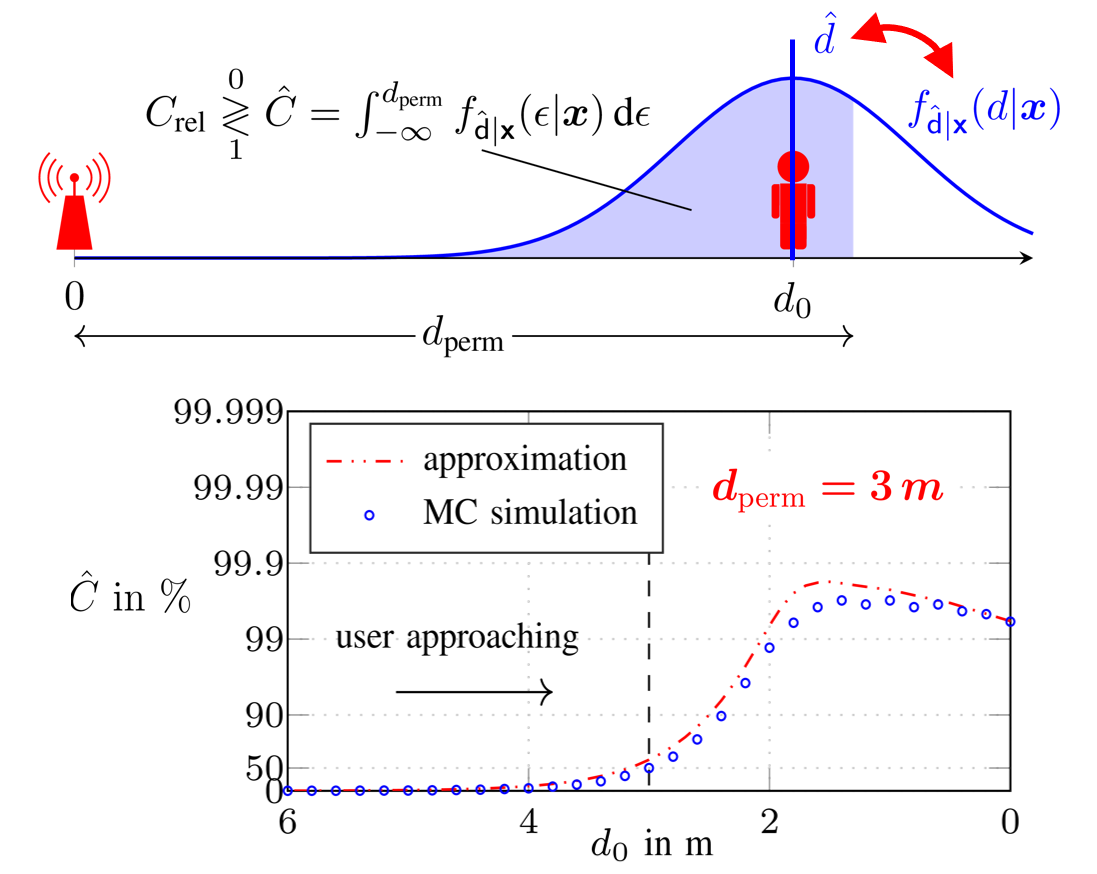Signal Processing and Speech Communication Laboratory

## Reliability and Threshold-Region Performance of TOA Estimators in Dense Multipath Channels

Published
Tue, Sep 01, 2020
Tags
rotm
ContactThis work, we investigate the reliability of time-of-arrival (TOA) based ranging using maximum-likelihood (ML) estimation in a dense multipath (DM) channel in terms of both the conventional mean squared error (MSE) as well as confidence bounds. We show that in the presence of DM the ML estimator distorts the signal mainlobe due to its whitening property, resulting in a bandwidth (BW) dependent bias, even before the outlier driven threshold region is reached.

Low-complexity metrics for accurately determining the performance in terms of the probability density (PDF) of the estimation error of both ML estimation and joint ML estimation and detection are provided. These metrics are based on the well known method of interval estimation (MIE) combined with local error prediction using the normalized noise-free likelihood (NNLIKE) and consider the non-Gaussian effects of outliers as well as mainlobe distortion.

The full version of the paper can be found on Researchgate

Figure: The upper graph shall depict the abstract use case. The anchor-agent distance d is bounded to a maximum permissible distance, by integrating over the PDF of the estimation error. A reliable algorithm yields an estimate together with the estimated confidence level (CL), which then is compared to a minimum reliable CL. The lower graph shows the estimated CL as a function of the actual distance using the presented approximation metric compared to results determined by the use of monte carlo (MC) simulation.

Browse the Results of the Month archive.